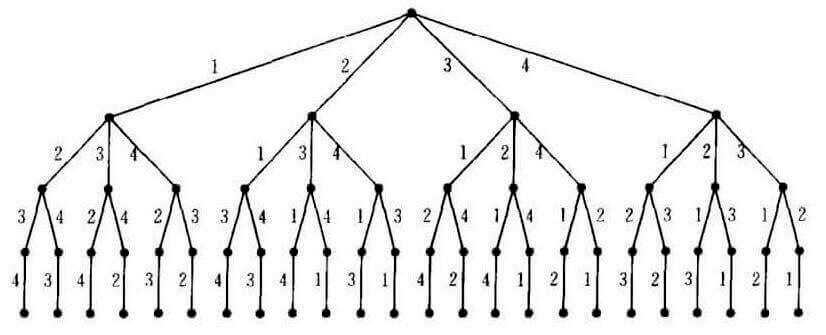# LeetCode 90. Subsets II

## Description

Subsets II

Given a collection of integers that might contain duplicates, nums, return all possible subsets (the power set).

Note: The solution set must not contain duplicate subsets.

Example:

``````Input: [1,2,2]
Output:
[
,
,
[1,2,2],
[2,2],
[1,2],
[]
]
``````

# LeetCode 79. Word Search

## Description

Word Search

Given a 2D board and a word, find if the word exists in the grid.

The word can be constructed from letters of sequentially adjacent cell, where “adjacent” cells are those horizontally or vertically neighboring. The same letter cell may not be used more than once.

Example:

``````board =
[
['A','B','C','E'],
['S','F','C','S'],
['A','D','E','E']
]

Given word = "ABCCED", return true.
Given word = "SEE", return true.
Given word = "ABCB", return false.
``````

`board`中查找是否存在相连（相邻）的字符与给定的单词对应。

# LeetCode 78. Subsets

## Description

Subsets

Given a set of distinct integers, nums, return all possible subsets (the power set).

Note: The solution set must not contain duplicate subsets.

Example:

``````Input: nums = [1,2,3]
Output:
[
,
,
,
[1,2,3],
[1,3],
[2,3],
[1,2],
[]
]
``````

# LeetCode 77. Combinations

## Description

Combinations

Given two integers n and k, return all possible combinations of k numbers out of 1 … n.

Example:

``````Input: n = 4, k = 2
Output:
[
[2,4],
[3,4],
[2,3],
[1,2],
[1,3],
[1,4],
]
``````

# LeetCode 60. Permutation Sequence

## Description

Permutation Sequence

The set `[1,2,3,...,n]` contains a total of n! unique permutations.

By listing and labeling all of the permutations in order, we get the following sequence for n = 3:

1. `"123"`
2. `"132"`
3. `"213"`
4. `"231"`
5. `"312"`
6. `"321"`

Given `n` and `k`, return the `k-th` permutation sequence.

Note:

• Given n will be between 1 and 9 inclusive.
• Given k will be between 1 and n! inclusive.

Example 1:

``````Input: n = 3, k = 3
Output: "213"
``````

Example 2:

``````Input: n = 4, k = 9
Output: "2314"
``````# LeetCode 40. Combination Sum II

Combination Sum II

## Description

Given a collection of candidate numbers (`candidates`) and a target number (`target`), find all unique combinations in candidates where the `candidate` numbers sums to `target`.

Each number in `candidates` may only be used once in the combination.

Note:

All numbers (including `target`) will be positive integers. The solution set must not contain duplicate combinations.

# LeetCode 39. Combination Sum

Combination Sum

## Description

Given a set of candidate numbers (`candidates`) (without duplicates) and a target number (`target`), find all unique combinations in `candidates` where the candidate numbers sums to `target`.

The same repeated number may be chosen from candidates unlimited number of times.

Note:

• All numbers (including `target`) will be positive integers.
• The solution set must not contain duplicate combinations.

# LeetCode 22. Generate Parentheses

Generate Parentheses

## Description

Given n pairs of parentheses, write a function to generate all combinations of well-formed parentheses.

For example, given n = 3, a solution set is:

``````[
"((()))",
"(()())",
"(())()",
"()(())",
"()()()"
]
``````Programer

ChengDu·China